# Sylow theorems

(diff) ← Older revision | Latest revision (diff) | Newer revision → (diff)

Three theorems on maximal-subgroups in a finite group, proved by L. Sylow  and playing a major role in the theory of finite groups. Sometimes the union of all three theorems is called Sylow's theorem.

Letbe a finite group of order, whereis a prime number not dividing. Then the following theorems hold.

Sylow's first theorem:contains subgroups of order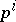for all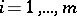; moreover, each subgroup of order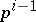is a normal subgroup in at least one subgroup of order. This theorem implies, in particular, the following important results: there is ina Sylow subgroup of order; any-subgroup ofis contained in some Sylow-subgroup of order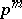; the index of a Sylow-subgroup is not divisible by; ifis a group of order, then any of its proper subgroups is contained in some maximal subgroup of orderand all maximal subgroups ofare normal.

Sylow's second theorem: All Sylow-subgroups of a finite group are conjugate.

For infinite groups the analogous result is, in general, false.

Sylow's third theorem: The number of Sylow-subgroups of a finite group divides the order of the group and is congruent to one modulo.

For arbitrary setsof prime numbers, analogous theorems have been obtained only for finite solvable groups (see Hall subgroup). For non-solvable groups the situation is different. For example, in the alternating group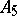of degree 5, for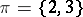there is a Sylow-subgroupof order 6 whose index is divisible by a number from. In addition, inthere is a Sylow-subgroup isomorphic to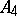and not conjugate with. The number of Sylow-subgroups indoes not divide the order of.

How to Cite This Entry:
Sylow theorems. Encyclopedia of Mathematics. URL: http://encyclopediaofmath.org/index.php?title=Sylow_theorems&oldid=11963
This article was adapted from an original article by V.D. Mazurov (originator), which appeared in Encyclopedia of Mathematics - ISBN 1402006098. See original article